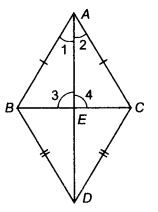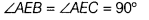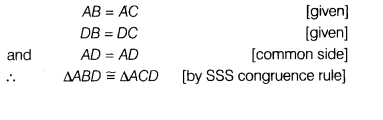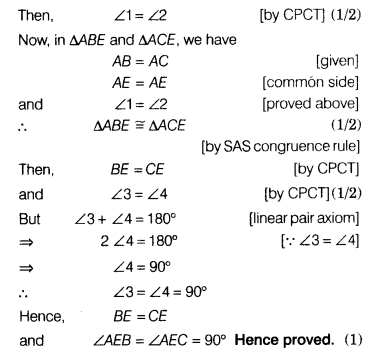# If two isosceles triangles have a common base, then prove that the line segment joining

If two isosceles triangles have a common base, then prove that the line segment joining their vertices bisects the common base at right angles.

Let ∆ABC and ∆DBC are the given triangles with same base BC, in which AB=AC and DB=DC.Also,Let AD meets BC at E.
To prove BE=CE andIn ∆ABD and ∆ACD,
we have